HomeUnderstanding AudioDouble amplifier power does not double the volume

# Double amplifier power does not double the volume

The relationship between amplifier power and volume is often misunderstood. This is partly due to terms like decibels, SPL, psycho-acoustics and other technical words. This article will unwrap this topic, explain some of these terms, let you discover some answers for yourself with an interactive exercise and also offers a calculator to help you understand the relationship of amplifier power, sound pressure and loudness.

### Decibels: on the level

A lot of confusion comes from the use of decibels, or rather the misuse of decibels. Decibels are used to simply describe the ratio of two levels. This might be two levels of power, two levels of voltage, two levels of sound pressure or two levels of loudness.

For a general introduction to decibels see the updated article on understanding decibels.

### Amplifier Power: watts this?

Amplifier power is the number of watts (measurement of power) a particular amplifier can produce (normally when it is flat out). For a better understanding of amplifier power and the different terms used to describe it, see Understanding Amplifier Power.  Typical ratings for amplifier power are: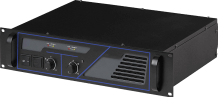• HiFi amplifiers:  20 watts to 100 watts
• Small PA systems: 30 watts to 250 watts
• Large sound systems: 100 watts to 3000 watts or more

Amplifier power corresponds to acoustic power. Doubling the amplifier power increases the acoustic power by 3dB. For example:

• Increasing an amplifier from 25 watts to 50 watts (double the power) increases the acoustic power by 3dB.
• Increasing an amplifier from 25 watts to 100 watts (4 times the power) increases the acoustic power by 6dB. This is same as doubling the power from 25 watts to 50 watt (3dB), and then doubling the 50 watts to 100 watts (another 3 dB), giving the total increase of acoustic power of 6 dB or 4 times the power.
• Increasing an amplifier from 25 watts to 250 watts (10 times the power) increases the acoustic power by 10dB.

The following table gives some more examples:

dB ChangePower change
+3x 2
+6x 4
+10x 10
+20x 100
+40x 10,000

However, please note that doubling the power does not double the Sound Pressure

### Sound Pressure:  it’s in the air

Acoustic power (produced by the amplifier) causes sound pressure waves to move in the air. This is commonly called sound pressure.  It is this sound pressure that our ears hear and microphones sense. The sound pressure is also what a sound pressure level (SPL) meter measures (in dBs). While the sound pressure is related to the acoustical power, doubling the acoustic power does not double the sound pressure.

• increasing the sound pressure by a factor of two (double) requires an increase of 6dB (x4) in amplifier power
• increasing the sound pressure by a factor of 10 (x 10) requires an increase of  20dB (x100) in amplifier power

The following table gives some more examples of these relationships:

dB ChangeSound pressure changePower change
+3x 1.41x 2
+6x 2x 4
+10x 3.16x 10
+20x 10x 100
+40x 100x 10,000

This tables show that:

• a 3dB increase in the amplifier power will double the acoustic power but only increase the sound pressure by a factor of 1.4
• a 6dB increase in the amplifier power will double the sound pressure, (and the power will be x 4)
• a 20dB increase in the amplifier power will give 10 times the sound pressure and 100 times the power

Note: While increasing the power level by a factor a four doubles the sound pressure, this doesn’t mean the volume is doubled.

### Volume or Loudness – it’s in the head

While acoustic power can be easily calculated and sound pressure can be easily measured, volume or loudness is subjective. It is the perceived level our ears and brain associate with different sound pressures. Volume level and loudness level are the same thing, I’ll call it loudness level. Loudness level will be perceived slightly differently by each person. It will also depend on the type of sound, the duration of the sound, how we feel, and all sorts of other subjective factors.

People who study these things are called psycho-acousticians. Most studies suggest that we need an increase of around 10dB for our hearing to perceive double loudness. Some studies suggest as low as 6dB.

What do you think? If your HiFi amplifier has a digital display which tells you the output volume in decibels, use your amplifier for the following exercise. If you don’t have access to a digital output display, you can use the following samples.

dB LevelSampleRelative to -20db
-20dB
0dB
-19dB
+1dB
-18dB
+2dB
-17dB
+3dB
-16dB
+4dB
-15dB
+5dB
-14dB
+6dB
-13dB
+7dB
-12dB
+8dB
-11dB
+9dB
-10dB
+10dB
• Set the level at -20dB (or click the -20dB play button above) and listen to the loudness.
• Move the volume to -17dB (or click the -17dB play button above). This is an increase of 3dB, which is double the power, but it doesn’t sound like double the volume to our ears.
• Move the volume to -14dB (or click the -14dB play button above). This is an increase of 6dB, which is 4 times the power and twice the sound pressure. This may sound like twice as loud as -20dB, or close to it.
• Continue to adjust the volume (or click different buttons above) and hear the level change. What level sounds twice as loud to you?
• What level sounds half as loud as -10dB to you?

As stated above, it is different for each person, and it depends on the duration,  type of music/noise and other factors. For the sample pink noise above, it may sound twice as loud anywhere from +6db to +10dB above the reference level of -20dB.

Whatever level you perceive as twice as loud, it is not at +3dB, which is twice the power. It is normally more than +6dB (4 times the power). Historically it is considered to be around +10dB, which is 10 times the power. This following table summarises the increases (the loudness is based on 10dB gain for double loudness).

dB ChangeLoudness ChangeSound Pressure ChangePower ChangeComments
+3x 1.23 (+23%)x 1.4x 2twice the power
+6x 1.52 (+52%)x 2x 4four times the power, twice the sound pressure
+10x 2x 3.16x 1010 x the power, around twice the loudness
+20x 4x 10x 100100 x the power, 10 x the sound pressure, around 4 times the loudness
+40x 16x 100x 10,000loud!

All this tells us that doubling the amplifier power does not double the perceived loudness or volume.

The percentage values are interesting. In general terms:

• Double the power (+3dB) gives around 25% increase in perceived volume
• Four times the power (+6dB) gives around 50% increase in perceived volume
• 10 times the power (+10dB) gives around 100% increase in perceived volume

To help you appreciate the difference a bigger amplifier may or may not have, use the following calculator. Simply input the first amplifier power and then the second amplifier power, and see the different levels of power, SPL and perceived loudness. The perceived loudness is based on the common understanding of requiring 10dB to perceive double loudness.

For those who need to know, the formulas used for these calculations are: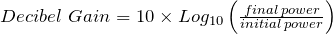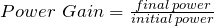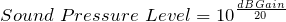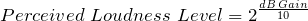And here is another formula, thanks to Earles L. McCaul, a reader from Tuscon, who pointed it out to me. With this formula you can calculate the perceived loudness increase when you increase amplifier power.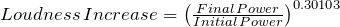For example if you have a 10 watts going to a speaker, and then increase the power to 100 watts: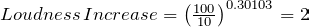That is, it will sound twice as loud.